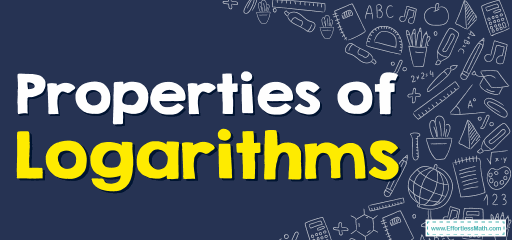# How to Use Properties of Logarithms? (+FREE Worksheet!)

Several logarithms properties help you solve logarithm equations. Here are some of them and their applications.## Necessary rules to solving Logarithm Equations

• Let’s review some logarithms properties:

$$a^{log_{a}{⁡b }}=b \ \ \ \ \ \ \ \ \ \ \ \ \ \ \ \ \ \ \ \ \ \ \ \ \ \ \ \ \ \ \ \ \ \ \ \ \ \ \ \ log_{a⁡}{\frac{1}{x}}=- log_{a}⁡{x}$$
$$log_{a}{⁡1}=0 \ \ \ \ \ \ \ \ \ \ \ \ \ \ \ \ \ \ \ \ \ \ \ \ \ \ \ \ \ \ \ \ \ \ \ \ \ \ \ log_{a⁡}{x^p}=p \ log_{a}⁡{x}$$
$$log_{a}{⁡a}=1 \ \ \ \ \ \ \ \ \ \ \ \ \ \ \ \ \ \ \ \ \ \ \ \ \ \ \ \ \ \ \ \ \ \ \ \ \ \ log_{x^k}{x}=\frac{1}{x} \ log_{k}⁡{x}$$ for $$k≠0$$
$$log_{a}{⁡(x.y)}=log_{a}{x} + log_{a}{y}\ \ \ \ \ \ \ \ \ \ log_{a⁡}{x}= log_{a^c}⁡{x^c}$$
$$log_{a}{⁡\frac{x}{y}}=log_{a}{x} – log_{a}{y} \ \ \ \ \ \ \ \ \ \ \ \ \ \ \ \ \ log_{a⁡}{x}=\frac{1}{log_{x}⁡{a}}$$

## Examples

### Properties of Logarithms – Example 1:

Expand this logarithm. $$log_{a}{(3×5)}=$$

Solution:

Use log rule: $$log_{a⁡}{(x .y)}=log_{a⁡}{x}+log_{a⁡}{y}$$
Then: $$log_{a}{⁡(3×5)}=log_{a }{3}+log_{a}{ 5}$$

### Properties of Logarithms – Example 2:

Condense this expression to a single logarithm. $$log_{a} {2}-log_{a }{7}$$

Solution:

Use log rule: $$log_{a}{x}-log_{a}{y}=log_{a}{\frac{x}{y}}$$
Then: $$log_{a}{2}-log_{a}{7}=log_{a}{\frac{2}{7}}$$

### Properties of Logarithms – Example 3:

Expand this logarithm. $$log(\frac{1}{7})=$$

Solution:

Use log rule: $$log_{a}{\frac{1}{x}}=-log_{a}{x}$$
Then: $$log(\frac{1}{7})= -log 7$$

### Properties of Logarithms – Example 4:

Condense this expression to a single logarithm. $$log_{a} {3}+log_{a }{8}$$

Solution:

Use log rule: $$log_{a}{x}+log_{a}{y}=log_{a⁡}{(x .y)}$$
Then: $$log_{a}{3}+log_{a}{8}=log_{a}{⁡(3×8)}=log_{a}{⁡24}$$

## Exercises for Properties of Logarithms

### Expand the logarithm.

1. $$\color{blue}{log(\frac{1}{5})=}$$

2. $$\color{blue}{log_{a}{(\frac{1}{2})}=}$$

3. $$\color{blue}{log_{a}{(2^5×8)}}$$

4. $$\color{blue}{log_{b}{(2x×7y)}}$$

### Condense into a single logarithm.

5. $$\color{blue}{log_{a}{x}+log_{a}{y}}$$

6. $$\color{blue}{log_{a}{2x}-2log_{a}{y}}$$

1. $$\color{blue}{-log 5}$$
2. $$\color{blue}{-log_{a} {2}}$$
3. $$\color{blue}{5log_{a} {2}+log_{a} {8}}$$
4. $$\color{blue}{log_{b} {2x}+log_{b} {7y}}$$
5. $$\color{blue}{log_{a} {xy}}$$
6. $$\color{blue}{log_{a} {\frac{2x}{y^2}}}$$

### What people say about "How to Use Properties of Logarithms? (+FREE Worksheet!) - Effortless Math: We Help Students Learn to LOVE Mathematics"?

No one replied yet.

X
30% OFF

Limited time only!

Save Over 30%

SAVE $5 It was$16.99 now it is \$11.99